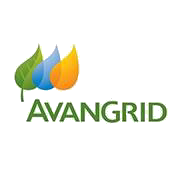# Avangrid Inc (NYSE:AGR)

46.12 USD +0.37 USD ( +0.81% )Avangrid Inc
NYSE:AGR

## DCF Value

Estimated DCF Value of oneAGR stock under the base case scenario is 37.29 USD. Compared to the current market price of 46.12 USD, the stock is Overvalued by 19%.

Estimated DCF Value of one NYSE:AGR stock is 37.29 USD. Compared to the current market price of 46.12 USD, the stock is Overvalued by 19% .

DCF valuation is one of two methods of placing a monetary value on a company; the other is Relative Valuation method. We use a combination of these two methods to calculate the Intrinsic Value of stock as accurately as possible.

Discount Rate
6.31%
Terminal Growth
0%
Growth Period
5 Years
Discount Rate
6.31%
Terminal Growth
0%
Growth Period
5 Years

You can change any inputs, such as future revenue, using Present Value Calculation block.

DCF Valuation FAQ:
AGR DCF Value
Base Case
37.29 USD
Overvaluation 19%
DCF Value
PriceAvangrid Inc Competitors:
DCF Valuation
AEP
American Electric Power Company Inc
JEL
Jersey Electricity PLC
PNW
Pinnacle West Capital Corp
CIG.C
Companhia Energetica de Minas Gerais CEMIG
DEIWY
Rio Paranapanema Energia SA

## DCF Value Calculation

### Capital Structure From Present Value to DCF ValuePresent Value 21.3B USD + Cash & Equivalents 1.5B USD + Investments 621M USD Firm Value 23.4B USD - Debt 8.1B USD - Minority Interest 885M USD Equity Value 14.4B USD / Shares Outstanding 387M AGR DCF Value 37.29 USD
Overvalued by 19%

To view the process of calculating the Present Value of Avangrid Inc' future free cash flow, see the Present Value Calculation block.

## Present Value Calculation

### Discounted Cash Flow Model Present Value of Free Cash FlowRotate your device for better experience.
Currency: USD

To view the process of deriving the DCF Value of one share from the estimated Present Value, see the DCF Value Calculation block.

## Sensitivity Analysis

### Sensitivity Analysis DCF Value Sensitivity AnalysisAnalyze the possible values of AGR stock DCF Value given various model inputs such as Revenue Growth, Operating Margin, and Discount Rate.

Similar Stocks

## AGR Stock DCF Valuation FAQ

What is the DCF value of one AGR stock?

Estimated DCF Value of oneAGR stock under the base case scenario is 37.29 USD. Compared to the current market price of 46.12 USD, the stock is Overvalued by 19%.

The true DCF Value lies somewhere between the worst-case and best-case scenario values. This is because the future is not predetermined, and the stock's DCF Value is based almost entirely on the future of the company. Knowing the full range of possible stock intrinsic values gives a complete picture of the investment risks and opportunities.

How was the DCF Value calculated?

1. Present Value Calculation. Using the DCF Operating Model we projectAvangrid Inc's future free cash flow and discount it at a selected discount rate to calculate its Present Value (21.3B USD).

2. DCF Value Calculation. We use the company's capital structure to calculate the total Equity Value based on the previously computed Present Value of the free cash flow. Dividing the Equity Value by the number of shares outstanding gives us the DCF Value of 37.29 USD per oneAGR share.